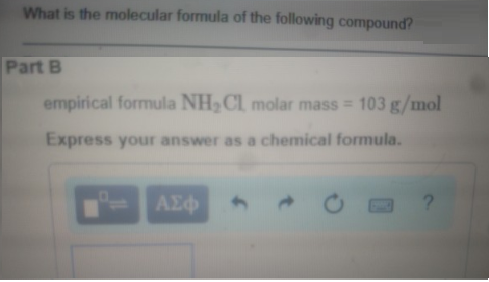# Problem: What is the molecular formula of the following compound? empirical formula NH2Cl molar mass 103 g/mol. Express your answer as a chemical formula.

###### FREE Expert Solution
80% (276 ratings)###### Problem Details

What is the molecular formula of the following compound?

empirical formula NH2Cl molar mass 103 g/mol.